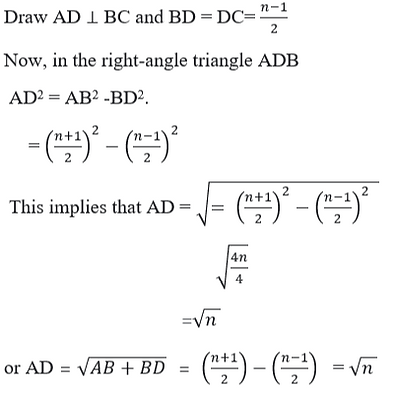top of page
##### Combining a large number of equal squares

The method of enlargement of elementary figures such as squares can be easily seen in the construction of Sara-Rathachakraciti (shape of a wheel with spokes) of Baudhayana. Similarly, Drona, citi, and many constructions require an enlargement of figures such as squares and rectangles. Datta (1932) specifies an auxiliary construction that must be made: 225 bricks laid out in a specific pattern that corresponds to the prescribed vedi area. Those are followed by an additional 64 identical bricks for a total of 289 in a square configuration. There are 289 square bricks in total (225 + 64), and they are organised in a square shape.  Baudhayana says,

“With these bricks, a square is to be formed. The side of a square (first formed comprises sixteen bricks.  Thirty–three bricks will still remain in excess. With this construction, the borders (on two sides) are completely round”. (Dutta,1932)

Katyayana Sulbasutra describes the methods of combining a large number of squares,

यावत्प्रमाणानि समचतुरस्त्राण्येकीकर्त्तुं चिकीर्षेदेकोनानि तानि भवन्ति तिर्यग्द्विगुणान्येकत एकाधिकानि त्र्यस्त्रिर्भवति तस्येषुस्तत्करोति||कात्यायन शुल्बसुत्रं ६.७ ||

“ As many squares (of equal area) as one wishes to combine, one should draw an isosceles triangle having the tiryanmani equal to the total length of all but on squares and twice a parsvamani,  equal to the total length of all plus one squares. The perpendicular of this triangle will combine all the squares”. (K.S. 6.7)

Let the side of the given square be one unit and ‘n’ be the number of squares. ABC be an isosceles triangle such that BC = n-1 and AB = AC = (n+1)/2